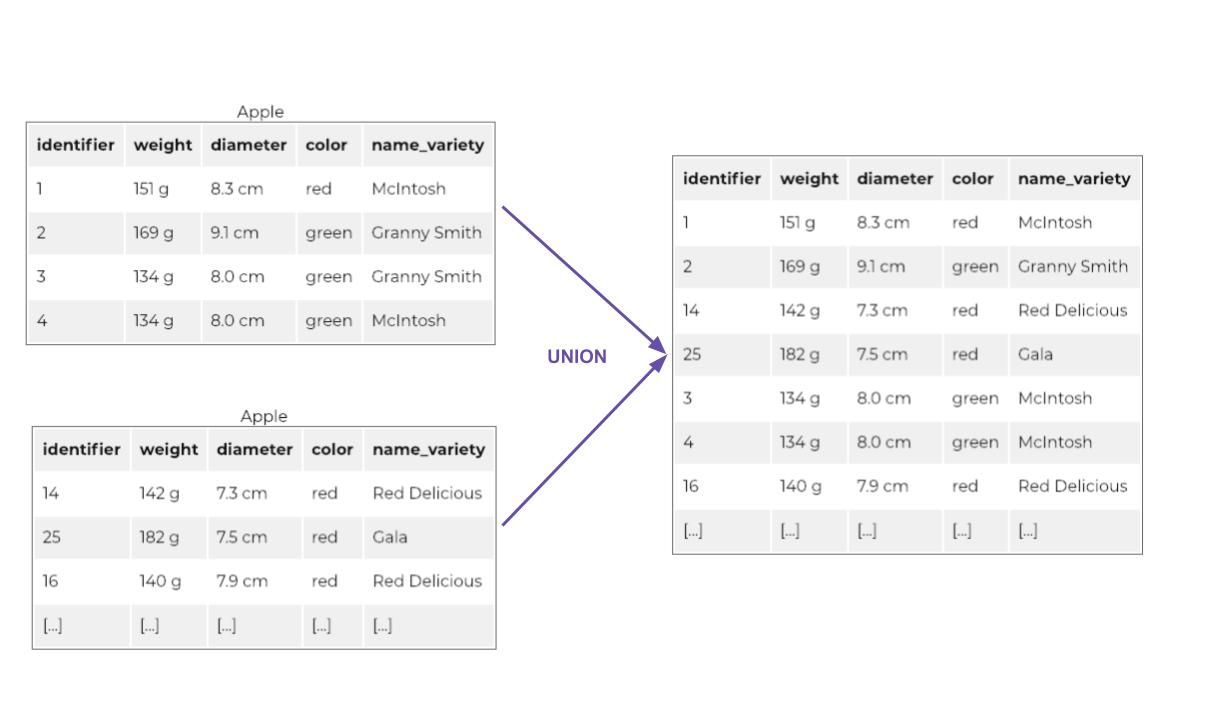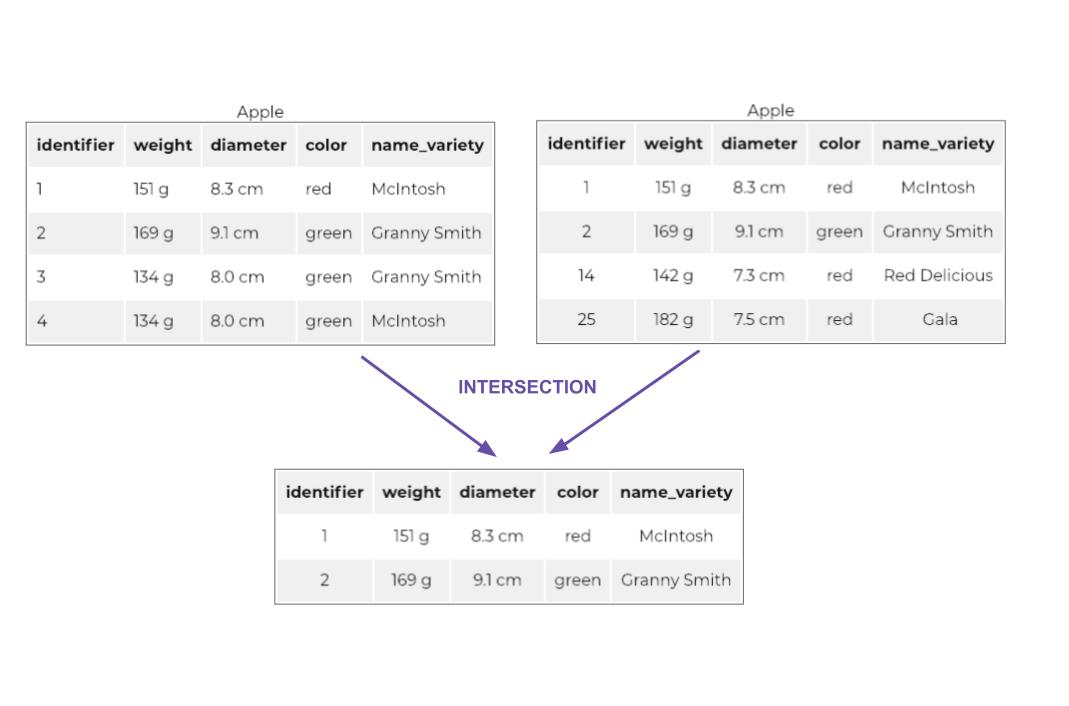Last updated on 4/19/21

## Combine relations using set operators

Log in or subscribe for free to enjoy all this course has to offer!Set operations are binary, meaning that they combine two (or sometimes more) elements to produce a third element. In relational algebra, set operators operate on pairs of relations contained within the same schema. In other words, set operations operate on a set of relations.

The simplest of the set operators has a rather poetic name: the Union.

Suppose we have two relations that represent apples. Let’s say each of these relations is a basket of apples. The union of these two baskets is achieved simply by pouring their respective contents into a third basket!

More formally :

The union of two relations of the same schema, R1 and R2, produces a third relation, also of the same schema, containing all of the tuples of R1 and R2Union

Difference is the opposite of union.

Difference is equivalent to subtracting apples from basket R3 and placing them in R2. The remaining apples of R3 are then moved to R1.  `R3 difference R2 = R1`.

The difference between an R3 and an R2 relation results in an R1 relation containing all of the tuples of R3 that are not found in R2.Difference

Finally, here is the third operator: intersection.

It is difficult to illustrate intersection with our example of baskets of apples. Why? Because in real life, one apple cannot be contained in two baskets at once. However, in relational algebra, the same tuple can indeed be contained in two different relations.

Here is the formal definition of intersection:

The intersection between two relations R1 and R2 results in a third relation containing only the tuples that are found in both R1 and R2.Intersection

For the three operations described in this chapter, I suggest the following notations:

`R1 union R2`

`R1 difference R2`

`R1 intersection R2`

• The union of two tables R1 and R2 contains all of the rows of R1 and R2.

• The difference between R3 and R2 contains all of the rows of R3 that are not found in R2.

• The intersection of R1 and R2 contains only the rows that are found in both R1 and R2.